Courses

# NCERT Exemplar - Kinetic Theory (Part- 1) Notes | EduRev

## JEE : NCERT Exemplar - Kinetic Theory (Part- 1) Notes | EduRev

The document NCERT Exemplar - Kinetic Theory (Part- 1) Notes | EduRev is a part of the JEE Course NEET Revision Notes.
All you need of JEE at this link: JEE

MULTIPLE CHOICE QUESTIONS  I

Q.1. A cubic vessel (with faces horizontal + vertical) contains an ideal gas at NTP. The vessel is being carried by a rocket which is moving at a speed of 500m s–1 in vertical direction. The pressure of the gas inside the vessel as observed by us on the ground
(a) Remains the same because 500m s-1 is very much smaller than vrms of the gas.
(b) Remains the same because motion of the vessel as a whole does not affect the relative motion of the gas molecules and the walls.

(c) Will increase by a factor equal to (v2rms + (500)2)/ v2rms where vrms was the original mean square  velocity of the gas.
(d) Will be different on the top wall and bottom wall of the vessel.
Ans.
(b)
Solution.
According to the ideal gas law,
P=nRT/V, here temperature of the vessel remain unchanged hence, the pressure remains same from that point of view.
Now, let us discuss the phenomenon inside the vessel. The gas molecules keep on colliding among themselves as well as with the walls of containing vessel. These collisions are perfectly elastic.
The number of collisions per unit volume in a gas remains constant. So, the pressure of the gas inside the vessel remains the same because motion of the vessel as a whole does not affect the relative motion of the gas molecules with respect to the walls.

Q.2. 1 mole of an ideal gas is contained in a cubical volume V , ABCDEFGH at 300 K (Figure). One face of the cube (EFGH) is made up of a material which totally absorbs any gas molecule incident on it. At any given time,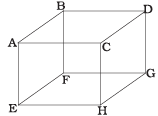(a) The pressure on EFGH would be zero.
(b) The pressure on all the faces will the equal.
(c) The pressure of EFGH would be double the pressure on ABCD.
(d) The pressure on EFGH would be half that on ABCD.
Ans.
(d)
Solution.
In an ideal gas, the gas molecules keep on colliding among themselves as well as with the walls of containing vessel. These collisions are perfectly elastic. So, their kinetic energy and momentum remains conserved.
So, the momentum transferred to the face ABCD = 2mv And the gas molecule is absorbed by the face EFGH. Hence it does not rebound. So, momentum transferred to the face EFGH = mv.
And the pressure on the faces is due to the total momentum to the faces. So, pressure on EFGH would be half that on ABCD.

Q.3. Boyle’s law is applicable for an
(b) Isothermal process.
(c) Isobaric process.
(d) Isochoric process.
Ans.
(b)
Solution.
Boyle’s law: For a given mass of an ideal gas at constant temperature, the volume of a gas is inversely proportional to its pressure.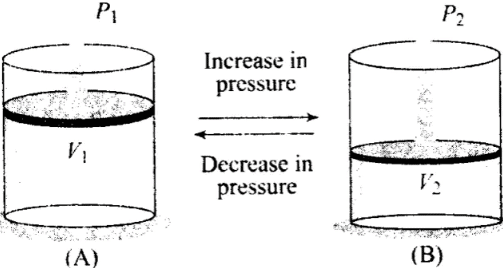So we can say that when temperature is constant, Boyle’s law is applicable.
i.e., PV= nRT= constant
⇒ PV = constant (at constant temperature)
i.e.. p ∝ 1/V - [where, P = pressure. V= volume]
So, this law is applicable for an isothermal process, in which temperature remain constant.

Q.4. A cylinder containing an ideal gas is in vertical position and has a piston of mass M that is able to move up or down without friction in Figure. If the temperature is increased,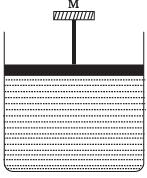(a) Both p and V of the gas will change.
(b) Only p will increase according to Charle’s law.
(c) V will change but not p.
(d) p will change but not V.
Ans.
(c)
Solution.
The situation is shown in the diagram where an ideal gas is contained in a cylinder, having a piston of mass M.
According to the problem, piston can move up and down without friction. So, the only force present is weight of the piston.
The pressure inside the gas will be
P = P0 + Mg/A = constant
where, P0 = atmospheric pressure
A = area of cross-section of the piston
Mg = weight of piston
Weight of piston and atmospheric pressure are constant, hence pressure remains constant.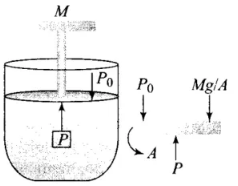According to ideal gas law,
PV = nRT
As the pressure remains constant, so if the temperature is increased, only the volume increases as the piston moves up without friction.

Q.5. Volume versus temperature graphs for a given mass of an ideal gas are shown in Figure, at two different values of constant pressure. What can be inferred about relation between P1 & P2?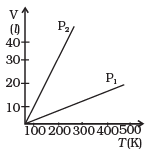(a) P1 > P2
(b) P1 = P2
(c) P1 < P2
(d) Data is insufficient
Ans
. (a)
Solution.
We have to consider slope of the V-T graph. From ideal equation, the slope of V-T curve is V/T = (nR/P).
So, if the slope is greater, pressure will be smaller and vice-versa.
We know for an ideal gas,
PV = nRT ⇒ V = (nR/P)T
Slope of the V-T graph, m = dV/dT = nR/P
⇒ m∝1/P [∴ nR = constant]
⇒ p ∝ 1/m
Hence, P1/P2 = m2/m1 < 1
So, P1 > P2.
where, m1 is slope of the graph corresponding to P1 and similarly m2 is slope corresponding to P2.
Hence the correct option is (a), i.e. P> P2

Q.6. 1 mole of H2 gas is contained in a box of volume V = 1.00 m3 at T = 300K. The gas is heated to a temperature of T = 3000K and the gas gets converted to a gas of hydrogen atoms. The final pressure would be (considering all gases to be ideal)
(a) Same as the pressure initially.
(b) 2 times the pressure initially.
(c) 10 times the pressure initially.
(d) 20 times the pressure initially.
Ans.
(d)
Solution.
The situation is shown in the diagram, H2 gas is contained in a box is heated and gets converted to a gas of hydrogen atoms. Then the number of moles would become twice.
According to gas equation,
PV= nRT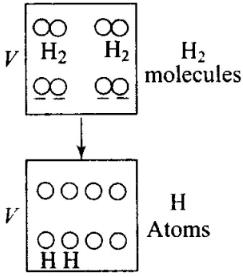P = Pressure of gas, n = Number of moles
R = Gas constant, T = Temperature PV = nRT
As volume (V) of the container is constant. Hence, when temperature (T) becomes 10 times, (from 300 K to 3000 K) pressure (P) also becomes 10 times, asP∝ T.
Pressure is due to the bombardment of particles and as gases break, the number of moles becomes twice of initial, so n2 = 2n1
So P ∝ nT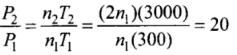⇒ P2 = 20P1
Hence, final pressure of the gas would be 20 times the pressure initially.

Q.7. A vessel of volume V contains a mixture of 1 mole of Hydrogen and 1 mole of Oxygen (both considered as ideal). Let f1 (v)dv, denote the fraction of molecules with speed between v and (v + dv) with f2 (v)dv, similarly for oxygen. Then
(a) F1(v) + f2 (v)= f (v) obeys the Maxwell’s distribution law.
(b) F1( v), f2 (v) will obey the Maxwell’s distribution law separately.
(c) Neither f1 (v), nor f2 (v) will obey the Maxwell’s distribution law.
(d) F2 (v)  and f1 (v) will be the same.
Ans.
(c)
Solution.
Maxwell’s Law (or the Distribution of Molecular Speeds):
1) The vrms gives us a general idea of molecular speeds in a gas at a given temperature. This doesn’t mean that the speed of each molecule is vrms. Many of the molecules have speed less than vrms and many have speeds greater than vrms.
(2) Maxwell derived equation gives the distribution of molecules in different speeds as follows: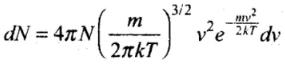where dN = Number of molecules with speeds between v and v + dv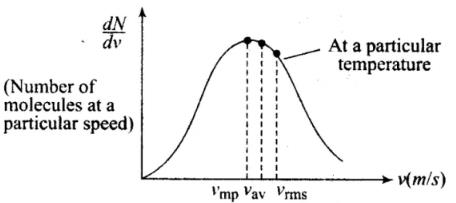The masses of hydrogen and oxygen molecules are different.

For a function f(v), the number of molecules dn = f[v), which are having speeds between v and v + dv. The Maxwell-Boltzmann speed distribution function ( Nv = dn/dv depends on the mass of the gas molecules.

For each function f1(v) and f2(v), n will be different, hence each function f1(v) and f2(v) will obey the Maxwell’s distribution law separately.

Q.8. An inflated rubber balloon contains one mole of an ideal gas, has a pressure p, volume V and temperature T. If the temperature rises to 1.1 T, and the volume is increased to 1.05 V,  the final pressure will be
(a) 1.1 p
(b) P
(c) Less than p
(d) Between p and 1.1
Ans.
(d)
Solution.
According to the equation of ideal gas, PV= nRT
P = pressure
V = volume
n = number of moles of gases
R = gas constant
T = temperature
Thus we have to rewrite this equation in such a way that no. of moles is given by,
n = PV/RT
As number of moles of the gas remains fixed, hence, we can write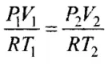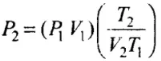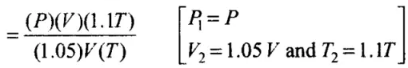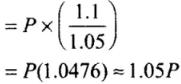Hence, final pressure P2 lies between P and 1.1 P.

MULTIPLE CHOICE QUESTIONS  II

Q.9. ABCDEFGH is a hollow cube made of an insulator (Fig.). Face ABCD has positve charge on it. Inside the cube, we have ionized hydrogen.
The usual kinetic theory expression for pressure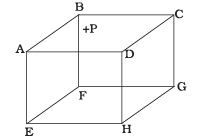(a) Will be valid.
(b) Will not be valid since the ions would experience forces other than due to collisions with the walls.
(c) Will not be valid since collisions with walls would not be elastic.
(d) Will not be valid because isotropy is lost.
Ans.
(b, d)
Solution.
According to the problem, ionized hydrogen is present inside the cube, they are having charge. Now, due to the presence of positive charge on the surface ABCD hydrogen ions would experience forces other than the forces due to collision with the walls of container. So, these forces must be of electrostatic nature. Hence, Isotropy of system is lost at only one face ABCD because of the presence of external positive charge. The usual expression for pressure on the basis of kinetic theory will be valid.

Q.10. Diatomic molecules like hydrogen have energies due to both translational as well as rotational motion. From the equation in kinetic theory pV = 2/3 E, E is
(a) The total energy per unit volume.
(b) Only the translational part of energy because rotational energy is very small compared to the translational energy.
(c) Only the translational part of the energy because during collisions with the wall pressure relates to change in linear momentum.
(d) The translational part of the energy because rotational energies of molecules can be of either sign and its average over all the molecules is zero.
Ans.
(c)
Solution.
According to kinetic theory equation, PV = 2/3 E [where P= Pressure V = volume]
E is representing only translational part of energy. Internal energy contains all types of energies like translational, rotational, vibrational etc. But the molecules of an ideal gas is treated as point masses in kinetic theory, so its kinetic energy is only due to translational motion. Point mass does not have rotational or vibrational motion. Here, we assumed that the walls only exert perpendicular forces on molecules. They do not exert any parallel force, hence there will not be any type of rotation present. The wall produces only change in translational motion.

Q.11. In a diatomic molecule, the rotational energy at a given temperature
(a) Obeys Maxwell’s distribution.
(b) Have the same value for all molecules.
(c) Equals the translational kinetic energy for each molecule.
(d) Is (2/3)rd the translational kinetic energy for each molecule.
Ans.
(a, d)
Solution.
Consider a diatomic molecule along z-axis so its rotational energy about z-axis is zero. So energy of diatomic molecule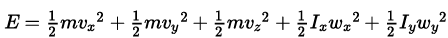(as moment of inertia along z axis is zero)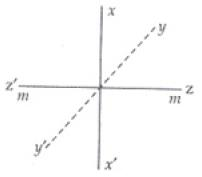The independent terms in the above expression is 5. As we can predict velocities of molecules by Maxwell’s distribution. Hence the above expression also obeys Maxwell’s distribution. As 2 rotational and 3 translational energies are associated with each molecule. So the rotational energy at given temperature is 2/3 of its translational Kinetic energy of each molecule.

Q.12. Which of the following diagrams (Figure) depicts ideal gas behaviour?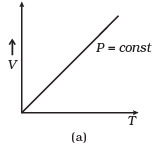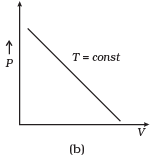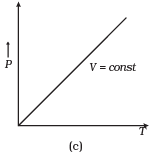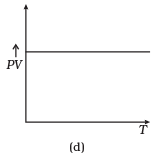Ans.
(a, c)
Solution.
For ideal gas behaviour,
PV = nRT ...(i)
(a) When pressure, P = constant
From (i) Volume V ∝ Temperature T
Graph of V versus T will be straight line.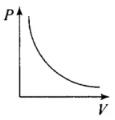(b) When T = constant
From (i) PV = constant
So, graph of P versus V will be a rectangular hyperbola. Hence this graph is wrong. The correct graph is shown below:
(c) When V = constant.
From (i) P ∝ T
So, the graph is a straight line passing through the origin.
(d) From (i) PV ∝ T
⇒ PV/T = constant
So, graph of PV versus T will be a straight line parallel to the temperature axis(x-axis).
i.e., slope of this graph will be zero.
So, (d) is not correct.

Q.13. When an ideal gas is compressed adiabatically, its temperature rises: the molecules on the average have more kinetic energy than before. The kinetic energy increases,
(a) Because of collisions with moving parts of the wall only.
(b) Because of collisions with the entire wall.
(c) Because the molecules gets accelerated in their motion inside the volume.
(d) Because of redistribution of energy amongst the molecules.
Ans.
(a)
Solution.
Since the gas is ideal and the collisions of the molecules are elastic. When the molecules collides with the moving parts of the wall, its kinetic energy increases. But the total kinetic energy of the system will remain conserved. When the gas is compressed adiabatically, the total work done on the gas increases, its internal energy which in turn increases the KE of gas molecules and hence, the collisions between molecules also increases.

Q.1. Calculate the number of atoms in 39.4 g gold. Molar mass of gold is 197 g mole-1.
Ans. Here, we know that,
Molar mass = Mass of Avogadro's number of atoms (Molecules)
= 6.023 x 1023 atoms.
According to the problem,
Mass of the gold, m = 39.4 g
Molar mass of the gold, M = 197 g mol-1
Now, 197 g of gold contains 6.023 x 1023 atoms
So, 1 g of gold contains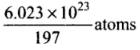∴ 39.4 g of gold contains (6.023 x 1023 x 39.4)/ 197 atoms = 1.20 x 1023 atoms

Q.2. The volume of a given mass of a gas at 27°C, 1 atm is 100 cc. What will be its volume at 327°C?
Ans.
By gas equation of ideal gas
P1 = 1atm, P2 = 1 atm, V1 = 100 cc, V2 = ?
T1 = 273 + 27 = 300 K T2 = 327 + 273 = 600K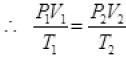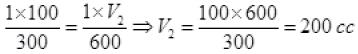Units of (P1, P2) and  (V1, V2) must be same separately but unit of T must be in only on Kelvin scale.

Q.3. The molecules of a given mass of a gas have root mean square speeds of 100 m s-1 at 27°C and 1.00 atmospheric pressure.What will be the root mean square speeds of the molecules of the gas at 127°C and 2.0 atmospheric pressure?
Ans.
vrms = 100 m/s
T1 = 27 + 273 = 300 K
v2rms = ?
T2 = 127 + 273 = 400K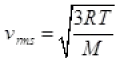M = Molar mass of gas for a gas M is constant
∴ vrms ∝ √T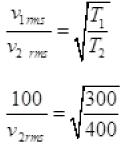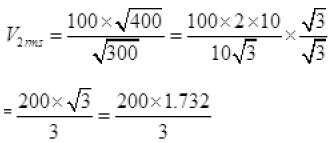v2rms =115.4 ms-1.

Q.4. Two molecules of a gas have speeds of 9 × 106 ms-1 and 1 ×106 ms-1, respectively. What is the root mean square speed of these molecules.
Ans.
RMS speed for w-molecules is defined as: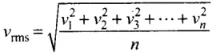[vrms = root mean square velocity]
where v1, v2, v1, ...vn are individual velocities of n - molecules of the gas.
For two molecules,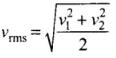According to the problem, v1 = 9 x 106 m/s and v2 = 1 x 106 m/s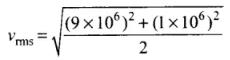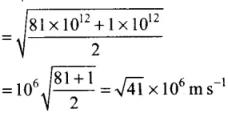Q.5. A gas mixture consists of 2.0 moles of oxygen and 4.0 moles of neon at temperature T. Neglecting all vibrational modes, calculate the total internal energy of the system. (Oxygen has two rotational modes.)
Ans.
Degree of Freedom:
The term degree of freedom of a system refers to the possible independent motions, systems can have or
The total number of independent modes (ways) in which a system can possess energy is called the degree of freedom (f).
The independent motions can be translational, rotational or vibrational or any combination of these.
So the degree of freedom are of three types:
(i) Translational degree of freedom
(ii) Rotational degree of freedom
(iii) Vibrational degree of freedom
General expression for degree of freedom
f = 3A - B; where A = Number of independent particles.
B = Number of independent restriction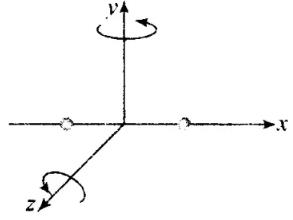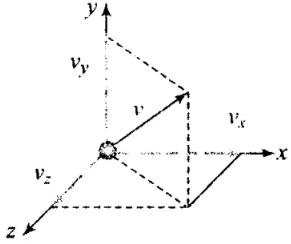Diatomic gas: Molecules of diatomic gas are made up of two atoms joined rigidly to one another through a bond. This cannot only move bodily, but also rotate about one of the three co-ordinate axes. However its moment of inertia about the axis joining the two atoms is negligible compared to that about the other two axes.
Hence it can have only two rotational motion. Thus a diatomic molecule has 5 degree of freedom: 3 translational and 2 rotational.
Monoatomic gas: Molecules of monoatomic gas can move in any direction in space so it can have three independent motions and hence 3 degrees of freedom (all translational).
According to the problem, n= 2 moles, nn = 4 moles
Temperature of the gas = T
Since, oxygen is a diatomic gas. So, degree of freedom associated with the molecules of the oxygen is 5.
Energy (total internal) per mole of the gas = 5/2 RT
[R = universal gas constant
T = temperature]
For 2 moles of the gas total internal energy = 2 x 5/2 RT = 5RT  ...(i)
Neon (Ne) is a monoatomic gas having 3 degrees of freedom.
Energy per mole = 3/2RT
Hence, Energy = 4 x 3/2 RT = 6RT ….(ii)
[Using Eqs. (i) and (ii)]
Total energy = 5RT = 6RT= 11RT

Q.6. Calculate the ratio of the mean free paths of the molecules of two gases having molecular diameters 1A and 2A . The gases may be considered under identical conditions of temperature, pressure and volume.
Ans.
Mean Free Path:
(1) The distance travelled by a gas molecule between two successive collisions is known as free path.
λ = Total distance travelled by a gas molecule between successive collisions / Total number of collisions During two successive collisions, a molecule of a gas moves in a straight line with constant velocity. Let λ1, λ, λ3 be the distance travelled by a gas molecule during n collisions respectively, then the mean free path of a gas molecule is given by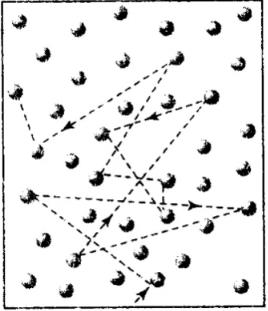(2) λ =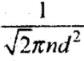where d = Diameter of the molecule,
n = Number of molecules per unit volume
According to the problem, d1 = 1 Å: d2 = 2Å
Since, mean free path,
λ ∝ 1/d2
⇒So,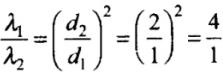Hence, λ1 : λ1 = 4 : 1
Neon (Ne) is a monoatomic gas having 3 degrees of freedom.
∴ Energy per mole = 3/2 RT
Hence, Energy = 4 x 3/2 (RT) = 6RT ...(ii)
[Using Eqs. (i) and (ii)]
Total energy = 5RT = 6RT = 11RT

Offer running on EduRev: Apply code STAYHOME200 to get INR 200 off on our premium plan EduRev Infinity!

,

,

,

,

,

,

,

,

,

,

,

,

,

,

,

,

,

,

,

,

,

;